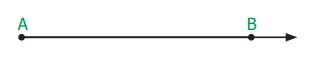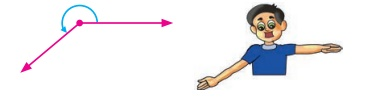Home | | Maths 7th Std | Geometry

# Geometry

Learning objectives ● To understand about adjacent angles, linear pair and vertically opposite angles. ● To understand transversal. ● To identify the different types of angles formed by a pair of lines wilth a transversal. ● To construct perpendicular bisector of a given line segment. ● To construct angle bisector of a given angle. ● To construct special angles such as 90°, 60°, 30° and 120° without using protractor.

Chapter 5

GEOMETRYLearning objectives

● To understand about adjacent angles, linear pair and vertically opposite angles.

● To understand transversal.

● To identify the different types of angles formed by a pair of lines wilth a transversal.

● To construct perpendicular bisector of a given line segment.

● To construct angle bisector of a given angle.

● To construct special angles such as 90°, 60°, 30° and 120° without using protractor.

Recap

Lines

Let us recall the following concepts on lines and points which we have learnt in class VI.

A line extends along both directions without any end. A line AB is denoted by. Sometimes small letters like l, m, n and so on are used to denote lines.A line segment has two end points. The line segment 'AB' is represented byA Ray is a line that starts from a point 'A' and extends without any end in a particular direction passing through 'B' which is denoted by.If two lines m and n are parallel, then we denote it as m||n. Parallel lines never intersect each other.When two lines have a common point they are called intersecting lines and that point is called the point of intersection of the given two lines.If three or more points lie on the same line, then they are called collinear points; otherwise they are called non-collinear points.Try these

1. Complete the following statements.

(i) A line is a straight path that goes on endlessly in two directions.

(ii) A line segment is a line with two end points.

(iii) A ray is a straight path that begins at a point and goes on and extends endlessly in the other direction.

(iv) The lines which intersect at right angles are perpendicular lines.

(v) The lines which intersect each other at a point are called Intersecting lines.

(vi) The lines that never intersect are called parallel lines.

2. Use a ruler or straightedge to draw each figure.

(i) line CD (ii) ray AB (iii) line segment MN3. Look at the figure and answer the following questions.(i) Which line is parallel to AB : GH

(ii) Name a line which intersect CD : IJ

(iii) Name the lines which are perpendicular to GH : KL

(iv) How many lines are parallel to IJ

one line KL

(v) Will EF intersect AB? Explain.

NO EF intersect KL

Angles

Recall that an angle is formed when two rays diverge from a common point. The rays forming an angle are called the arms of the angle and the common point is called the vertex of the angle. You have studied different types of angles, such as acute angle, right angle, obtuse angle, straight angle and reflex angle, in class VI. We can summarize them as follows:

Acute angle

An angle whose measure is less than 90º is called an acute angle.Right angle

An angle whose measure is exactly 90º is called a right angle.Obtuse angle

An angle whose measure is greater than 90º is called an obtuse angle.Straight angle

An angle whose measure is exactly 180° is called a straight angle.Reflex angle

An angle whose measure is greater than 180° and less than 360° is called a reflex angle.

Also, we have studied about related angles such as complementary and supplementary angles in our previous class. Let us recall them.Complementary angles

Two angles are called Complementary angles if their sum is 90°.Are the two angles given in the figure complementary? Yes.

The pair of angles 35° and 55° are complementary, where the angle 35° is said to be the complement of the other angle 55° and vice versa.

Supplementary angles

Two angles are called Supplementary angles if their sum is 180°.Observe the sum of measures of the angles 70° and 110° given in the figure is 180°. When two angles are supplementary, each angle is said to be the supplement of the other.

Try these

1. A straight angle measures

(a) 45°

(b) 90°

(c) 180°

(d) 100°

2. An angle with measure 128° is called ____________ angle.

(a) a straight

(b) an obtuse

(c) an acute

(d) Right

3. The corner of the A4 paper has

(a) an acute angle

(b) a right angle

(c) straight

(d) an obtuse angle

Answer : (b) a right angle

4. If a perpendicular line is bisecting the given line, you would have two

(a) right angles

(b) obtuse angles

(c) acute angles

(d) reflex angles

5. An angle that measure 0° is called _______.

(a) right angle

(b) obtuse angle

(c) acute angle

(d) zero angle

Introduction

We are familiar with complementary and supplementary angles. Let us see some more related pairs of angles now.

MATHEMATICS ALIVE - GEOMETRY IN REAL LIFEBeauty of Angles in architecture and kolams

Tags : Term 1 Chapter 5 | 7th Maths , 7th Maths : Term 1 Unit 5 : Geometry
Study Material, Lecturing Notes, Assignment, Reference, Wiki description explanation, brief detail
7th Maths : Term 1 Unit 5 : Geometry : Geometry | Term 1 Chapter 5 | 7th Maths